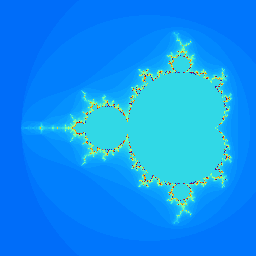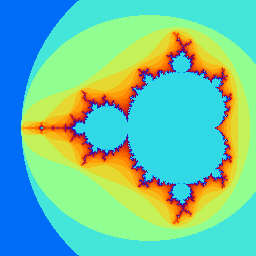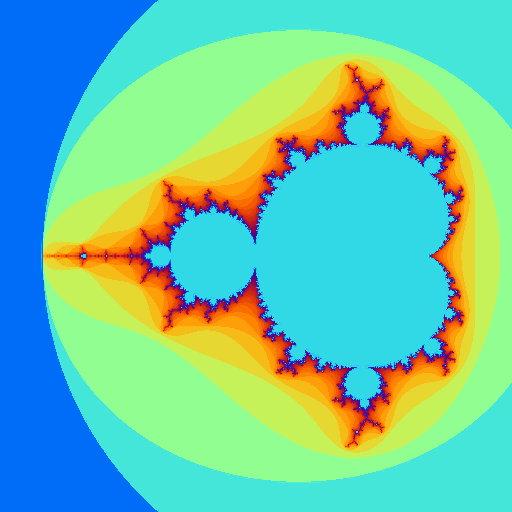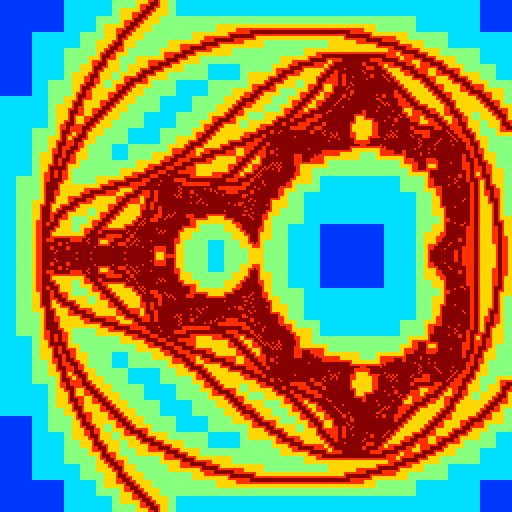# sandbox/Antoonvh/mandelbrot2.cThe natural beauty of the Dettifoss waterfall may be rooted in the wide range of length scales that can be observed. Image via Ontdek IJsland.nl

# Introduction

Nature seems to organize itself so that a variety of objects exist and more often than not, these are characterized with an internal spatial structure that appears over wide range of scales. For some processes in nature, the structures that occur at the different scales are related to each other via a characteristic fractal pattern. As a toy model for fractals, Benoit B. Mandelbrot came up with a simple recipe to create a mathematical object with a spectacular non-selfsimilar character. On this page we aim to visualize its shape using an adaptive grid.

## Visualizing the Mandelbrot set

For a complex number c, one can define the sequence:

\displaystyle z_{n+1} = z_{n} ^2 + c,

starting from z_0=c. It can be shown that when \|z_n\|>2 the sequence diverges in an absolute sense and the Mandelbrot set is the set of complex numbers for which the aforementioned squence does not diverge. A worthwhile visualization occurs when we draw the complex plane and colour code each pixel with to the number of iterations (N) it takes such that \|z_N\|>2, using the pixel’s-centered location as c. As such we include a 2D grid, some utilities, define a color bar and write a function that returns N for the complex number c= x_p + iy_p, with i = \sqrt{-1}.

#include "grid/quadtree.h"
#include "utils.h"

void rainbow (double cmap[NCMAP]){
for (int i = 0; i < NCMAP; i++){
cmap[i] = sq(sin((double)i*M_PI/110.));
cmap[i] = sq(sin(((double)i + 25.)*M_PI/110.));
cmap[i] = sq(sin(((double)i + 50.)*M_PI/110.));
}
}

double Nmax;
double N_iters (double xp, double yp){
int j = 0;
double ab = (sq(xp) + sq(yp));
double a = xp;
double b = yp;
double c;
while (j <= Nmax && ab < 4){
c = (a*a) - (b*b) + xp;// Real part
b = (2.*a*b) + yp; //Imag part
a = c;
j++;
ab=((a*a) + (b*b));
}
return (double)j;
}

This is sufficient for a 256 \times 256 pixels visualization for a c where -2.25<\text{Re}(c)<1.25 and -1.5<\text{Im}(c)<1.5. Also we stop checking for divergence when n > 100.

scalar it[];
int main(){
L0 = 3.;
X0 = -2.25;
Y0 = -L0/2;
init_grid (256);
Nmax = 100;
foreach()
it[] = N_iters (x, y);
output_ppm (it, file = "firstimage.png", map = rainbow,
min = 0, max = (double)Nmax);

The result looks like this:a 256\times256 rendering of the Mandelbrot set.

We do recognize the famous set, but the visualization is not very statisfactory. We should colour code the pixels according to the logarithm of the number of iterations N.

  foreach()
it[] = log (it[] + 1.);
output_ppm(it, file = "secondimage.png", map = rainbow,
min = 0, max = log ((double)Nmax + 1.));

This is the result:Another 256\times 256 rendering of the Mandelbrot set.

Much better!

One may be curious enough to inspect the smaller scale features of the set, however the 256 \times 256 pixels rendering can only display so much detail. Therefore we should increase the resolution. However, we can already see that a large fraction of the domain does not contain any interesting features. As such we only wish to calculate the colour codes at a high resolution where it is required and use straight forward linear interpolation for the locations where the colours vary smoothly.

At this moment we realize that we can only display the set with a finite colour coding accuracy. For this case, there are only NCMAP number of colours in our palette. The value is which (i.e. 127) is chosen large enough so that it does not affect the visual appearance too much. We accept the fate regarding our discretized approach and embrace it to formulate a grid adaptation strategy. In order to detect where the rendering is smooth, we check if the colour coding of a cell can be reproduced accurately enough by linear interpolating from a coarser grid. Alternatively, we detect the presence of “features” that require refinement if we are unable to reconstruct a local colour code from a coarser grid rendering. Only when the reconstruction falls within a small range of accaptable colour tolerance we keep the grid as is. In order to prevent indefinite refinement we limit the algorithm to refine upto a maximum resolution that corresponds to the resolution of our desired image.

It makes sense to set the error threshhold to be 1/NCMAP, as we have identified this as an accaptable colour error for our eyes. Fortunately we can conviniently implement the above algorithm using wavelet thresholding. We use the following code to implement it and render an 512 \times 512 image.

  void coarsen_mandelbrot(Point point, scalar s){
s[] = log (N_iters (x, y) + 1.);
}
it.coarsen = it.restriction = coarsen_mandelbrot;
unrefine (level > 6);
foreach()
it[] = log (N_iters (x, y) + 1.);
boundary({it});
}
output_ppm(it, n = 512, file = "thirdimage.png", map = rainbow,
min = 0, max = log ((double)Nmax + 1.), linear = true);

Here is the result:A 512\times 512 rendering of the Mandelbrot set.

It looks OK, we also render the used grid:

  scalar lev[];
foreach()
lev[] = level;
output_ppm (lev, n = 512, file = "level.png", min = 3, max = 9);

We colour code the grid according to the local level of refinement.The levels of the used quadtree grid for the 512\times512 rendering.

Before we push to even higher resolutions, we check if our algorithm did a good job by comparing our result against a 512 \times 512 equidistant approach. We compare the color codes of our interpolated pixels against their ‘true’ value. We make a histogram of the actual error using 256 equally-spaced bins ranging from 0 to 4/NCMAP.

  int errors = {0};

refine (level < 9);//Linear interpolation.
foreach(){
int err_index = (int)(256.*fabs(it[] - log (N_iters (x, y) + 1.))/4.);
if (err_index < 256)
errors[err_index]++;
}
FILE * fp = fopen ("hist_errors", "w");
for (int j = 0; j < 256; j++)
fprintf (fp, "%g\t%d\n", 4.*(double)j/256., errors[j]);
fclose (fp);

We plot the histogram data:

set yr [1:10000]
set ylabel 'count'
set xlabel 'error [colour-code index]'
set logscale y
set key off
plot 'hist_errors' u 1:2(script)

There exist no pixels with an error larger than our criterion.

## Fractal scaling

The adapted grid that we have used for our renderings has enherited some of the internal structure of the object that we are studying. A not-so-obvious feature arrises when we study the number of used grid cells as function of our resolution. Starting from a 128 \times 128 grid, we iteratively double the resolution up to a 16384 \times 16384 equivalent and track the used number of grid cells.

  int cells = {0};
unrefine (level > 5);
for (int maxlevel = 6; maxlevel <= 14; maxlevel++){
foreach()
it[] = log (N_iters (x, y) + 1.);
boundary ({it});
}
foreach()
cells[maxlevel - 6]++;
}
FILE * fp2 = fopen ("cells", "w");
for (int maxlevel = 6; maxlevel <= 14; maxlevel++)
fprintf (fp2, "%d\t%d\n", maxlevel, cells[maxlevel - 6]);
fclose (fp2);

We plot the resulting number of cells :

set xr [ 5.5:14.5]
set yr [ 1000:25000000]
set xlabel 'Level'
set ylabel 'Cells'
set key box top left
plot 'cells' u 1 : 2 t 'Data' ,\
10*2**(x*1.5) w l t '1.5D scaling'(script)

Remarkably, for this purpose there appears to be a characteristic scaling behaviour that is maintained over a wide range of scales. The equidistant approach would require calculations on 16384 \times 16384\approx 2.7\times10^8 cells and we have reduced it by a factor of 20. This reduction factor increases further for larger images. As such we were enabled to render a 131072 \times 131072 pixels image of the set. Feel free to explore it via this link:

16 Giagapixel rendering of the Mandelbrot set

To faciliate the rendering with 17 levels of refinement, we used a modified colour bar, Nmax = 2000, a modified version of the output_ppm() function and the PPM was converted to a PNG image offline, using convert.

}